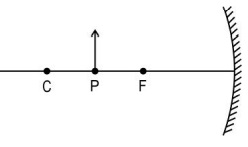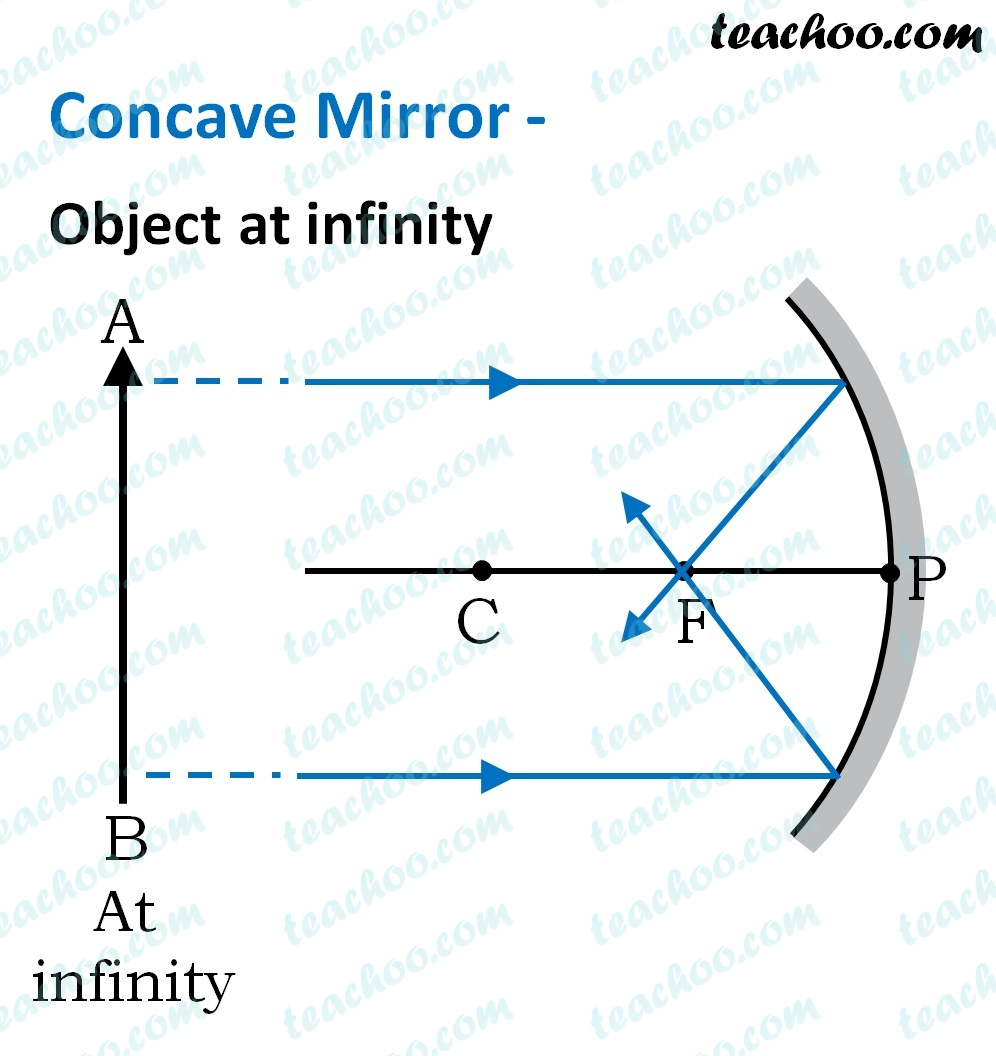Practice Questions CBSE - Science Class 10 (2023 Boards)

Class 10
Solutions to CBSE Sample Paper - Science Class 10

## An object of height h is kept at point P in front of a mirror as shown below. The height of the image produced is h'. In the diagram, F is the focus and C is the centre of curvature.## (b) If the focal length of the mirror is 20 cm and the distance between points P and C is 10 cm, determine the distance between the images produced when the object is kept at P and C.

(a) M = H’/H

• Image produced in the given case (object between C and F) is greater than the object in sizeTherefore, M>1.

• When object is at C, image is also produced at C.

H’=HTherefore, M=1.

Thus the height of the image produced when the object is at C will be less than the image produced when object is between C and F.

(b)

To find the distance between the two images we need to find the image distance when the object is at P and when it is at C.

• To find the image distance when the object is at P

u = -30 cm

f = -20 cm

• Using mirror formula :
1/f=1/u+1/v
• v1 = -60 cm
• To find the image distance when the object is at C :
Since C is the centre of curvature,
image distance = object distance (i.e.)
• v2 = - 40 cm

Distance between the images = |v2| - |v1| = 60 - 40 = 20 cm

Learn in your speed, with individual attention - Teachoo Maths 1-on-1 Class